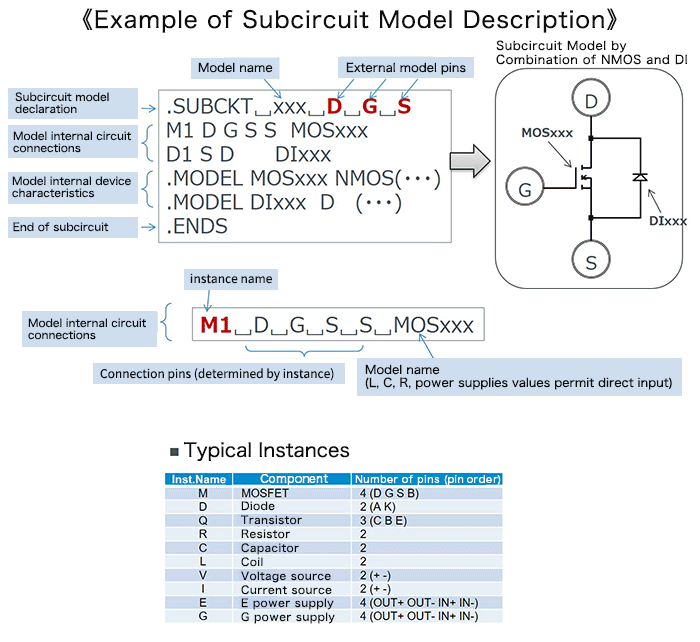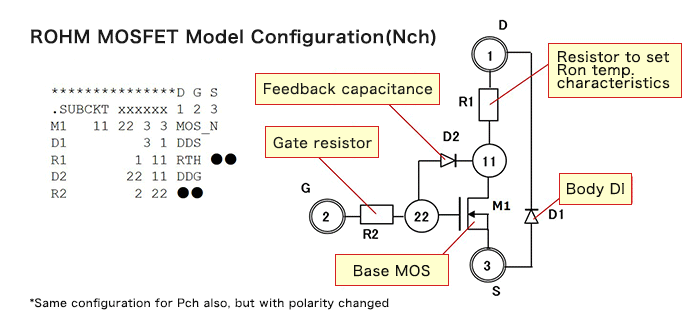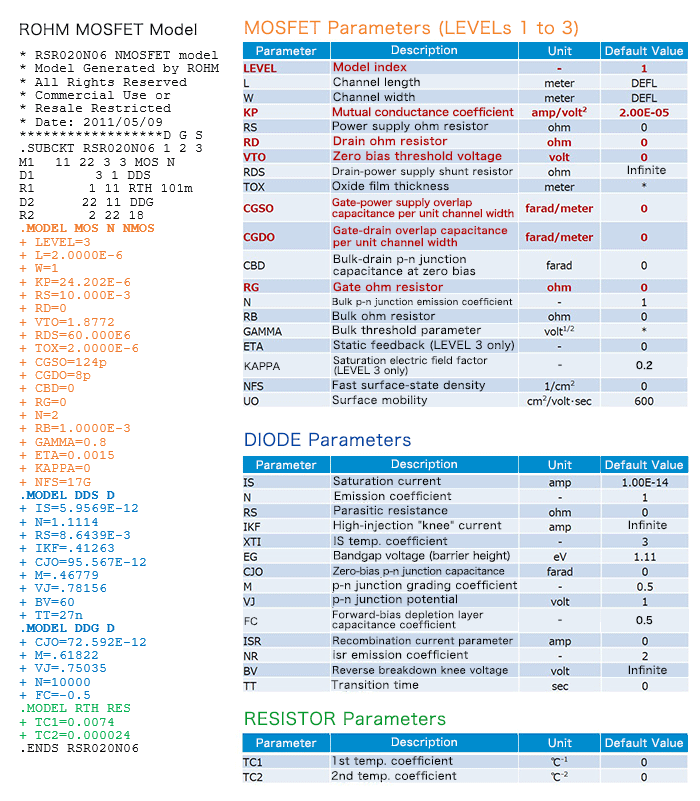# SPICE Subcircuit Models: MOSFET Example―Part 1

2019.06.20

・There are two types of SPICE models: “device models” and “subcircuit models”.

・Subcircuit models consist of connection information and device models.

・Subcircuit models may be constructed by imparting realistic characteristics to device models that represent ideal characteristics, and may constitute circuits with specific functions or the like.

In the previous article and in the article before that, examples of SPICE device models were explained. In this article and the next, SPICE subcircuit models are explained, using MOSFETs as examples.

## SPICE Subcircuit Models (Review)

The basic configurations of device models and subcircuit models were explained in SPICE Model Types, but subcircuit models are somewhat complicated, and so are here briefly reviewed.
Subcircuit models are models of circuits, and such models include circuit connection information, device models, and other information.In the above example of a subcircuit model that combines an Nch MOSFET and a diode, the circuit connections, a device model of the MOSFET, and a device model of the diode are described. In the “model internal circuit connections”, an instance name is followed by connection terminals and the model name. In this model, the connection of the MOSFET M1 and the diode D1 is described.

### Actual Subcircuit Model Example

In the previous explanations, simple models were used to facilitate understanding; here an actual Subcircuit model is used in explanations. The following is a description of the circuit and circuit connections of a subcircuit model for a ROHM Nch MOSFET. In the previous example, the device model for a MOSFET labeled “M1” appeared. The reader will be wondering why we are considering a “MOSFET subcircuit model” when a device model was previously described. This is because a device model is essentially an ideal model, whereas in order to represent the actual MOSFET characteristics, what is often actually used is a subcircuit model, obtained by adding parasitic components, temperature characteristics, and the like to a MOSFET device model that serves as the base. Of course, there are also many subcircuit models of for example circuits in which the components necessary for a power supply IC are connected and the circuit is operated as a power supply, but these would be too complex to use as examples here, and so an example of a MOSFET subcircuit model was selected.To begin with, examine the descriptions of connections from M1 to R2, and the node numbers in the circuit diagram. The meanings of the descriptions should be immediately understandable.

This subcircuit model is a SPICE model that represents characteristics close to those of an actual MOSFET by adding, to the MOSFET M1 serving as the base model, a feedback capacitance, gate resistance, body diode, and resistance that imparts the temperature characteristic of the on-resistance Ron.

The following is the complete description of the subcircuit model. In addition to the connection information described above, there are descriptions of device models for the MOSFET, diode, and resistances, constituting the subcircuit model. The table lists parameters of each device model.It should also be noted that in order to create an excellent SPICE model, that is, a SPICE model that represents realistic characteristics, it is necessary to know just what subcircuit models to incorporate. These differ depending on the model source, but it will probably be necessary to confirm the quality of the model, by for example comparing simulation results with actual characteristics.

(Continued to Part 2)